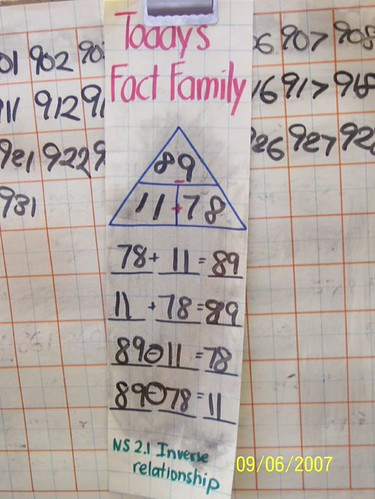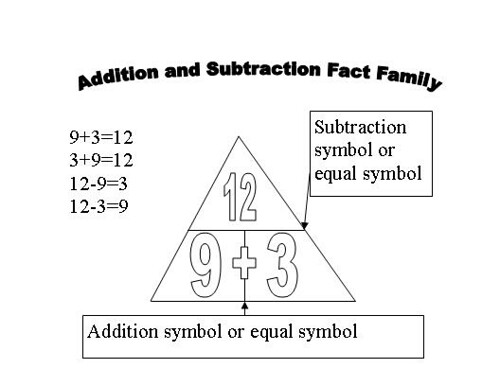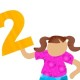## Using Triangles to Teach Fact Family

September 9, 2007 at 4:01 pm | Posted in best practices, elementary, graphic organizers, math, strategies | 2 Comments

For the past 7 weeks, I have used this modified triangle as a graphics organizer, originally developed by Andrew, to teach my second grade students math fact families. Fact family is part of our daily math activities. For about five minutes every day, we look at one new fact family and explore relationships between the members of the fact family. Here’s a picture of the latest fact family that we are working on.As you can see, it’s just a laminated piece of paper that I reuse every day.

### Success

Our math assessment, which we took just last week, showed me how incredibly successful using the triangle is in teaching fact family and many relationships that are embedded in fact family. I’ll give you the hard numbers when the scores come back from the district, but a teacher look-see showed me that almost all of the students got all of the related fact family questions correct.

### Daily Math

My students and I do what we call “Daily Math”. We break up our one hour of math time into two parts. We spend 20 minutes a day on daily math and 40 minutes on a new lesson. Currently, daily math contains counting time, which I will blog about later, “Today’s Fact Family”, patterns, and one word problem. We do daily math as a whole group on the carpet. Daily math is where I build number sense and practice key concepts.

### What a teacher should know

Fact family isn’t just a question on a math test that keeps cropping up. It contains important mathematical concepts: inverse relationship, commutative and anticommutative property of addition and subtraction, property of equality, missing addends, and of course basic math facts. Fact family is of practical use in that all a student needs to know is one fact family member to know all four related facts, saving time. But in order to use this important concept, students need to understand fact family further than simply one lesson can provide. They need daily practice until it becomes automatic. For my students, it has become automatic.

### How I Introduced Fact FamilyI explicitly tell me students that knowing your fact family will save time doing addition and subtraction problem because if you know one fact, you know four facts. Also, it is helpful in helping to check your answers. For me, it is always important to tell a student why they are learning something, that there is always a purpose to motivate the learning. Part of clear expectations.

Then we do the first fact family together. Since it is the first time, I wrote three numbers in the triangle, 5-3-2. I showed them how to read the triangle, going this way and that way. We read the triangle together, then I showed them how to write the related number sentences. Next, we check to make sure that we weren’t writing down nonsense number sentences. We use our fingers to check EACH number sentence to verify that EACH number sentence is correct. I ask my students, “Does this work?” The answer is yes. We are then done with fact family for the day.

### Subsequent Lessons

For a few more lessons after that, until students become comfortable with reading the triangle and writing the number sentences, we do just that. Then, as students become comfortable, at the end of the lesson, I would change a number in one of the number sentences, perhaps an addend or the sum or the difference. We would check the number sentence to see if the number sentence is still correct. The number sentence would not be correct, further reinforcing the idea of the fact family, that only these three numbers can exist in one fact family.

### Missing Numbers

A few lessons after that, I would start to leave off a number in the triangle, creating a missing addend or a missing sum/difference. We would read the triangle thus: “2 plus blank equals 5, blank plus 2 equals five, five minus blank equals 2, five minus 2 equals blank”. This is where things get exciting. Students who are developing a fine sense of number concept and fact family would immediately recognize that if they can solve one equation, then they have the missing number to all of them. For students who don’t have that fine number sense, doing this activity over and over again helps build it for them.

### Check this Number Sentence

In later lessons, we would start to “check” a number sentence. Instead of starting at the triangle, I would give the student one number sentence “13-8=5”. I would ask them to find another number sentence that would help me check this number sentence. The students usually struggle at first, but someone would recognize that they can use fact family to check the number sentence. Then, we would fill in the triangle, pull our related fact family members and choose the two addition sentences to check our number sentence. Many teachers can see that how useful this is now because math tests are just LITTERED with “check the number sentence” type questions.

### Non-examples

Also, through out all these lessons, I would throw in a non-example. Non-examples are useful to examine. Developing a sense of what’s right is great, but without a sense of what’s wrong, you haven’t fully developed understanding. For example, in checking a number sentence, I would give the student a number sentence that is completely wrong like “13-8=6”. At first, the student will give me this fact family “13-8-6” and say that “8+6=13” and “6+8=13” can be used to check the number sentence. I ask, are you sure? Confusion for a moment, then someone will discover that 8+6 can never equal 13 and the student will demonstrate that 8+6=14 so the original subtraction sentence is incorrect. THIS is how we learn the usefulness of fact family. I was very happy when my students get to this point. From then on, when I give them an incorrect fact to check, they pull out a related fact family, choose an easy one to check, and then go for it.

### Inverse Relationship

Weeks have passed by now. My students are quite comfortable with fact family, the language of fact family. We did a fantastic job passing our math test, yadiyadiya. I look at some questions that might be on the next math test and discover the question, “What is the inverse of _____?” Now, it’s simple for me to go back, tell the students that inverse means the opposite, that if they give you an addition sentence, they want a related subtraction sentence. Now, we add “What is the inverse of ___?” to our daily fact family practice. I’m sure my students will do fine on that portion of the test next as well. It’s a concept they already know, now they have the language for it to pass the test.

1.2.